# An introduction to cutpointr

#### 2021-06-29

cutpointr is an R package for tidy calculation of “optimal” cutpoints. It supports several methods for calculating cutpoints and includes several metrics that can be maximized or minimized by selecting a cutpoint. Some of these methods are designed to be more robust than the simple empirical optimization of a metric. Additionally, cutpointr can automatically bootstrap the variability of the optimal cutpoints and return out-of-bag estimates of various performance metrics.

## Installation

You can install cutpointr from CRAN using the menu in RStudio or simply:

install.packages("cutpointr")

## Example

For example, the optimal cutpoint for the included data set is 2 when maximizing the sum of sensitivity and specificity.

library(cutpointr)
data(suicide)
head(suicide)
##   age gender dsi suicide
## 1  29 female   1      no
## 2  26   male   0      no
## 3  26 female   0      no
## 4  27 female   0      no
## 5  28 female   0      no
## 6  53   male   2      no
cp <- cutpointr(suicide, dsi, suicide,
method = maximize_metric, metric = sum_sens_spec)
## Assuming the positive class is yes
## Assuming the positive class has higher x values
summary(cp)
## Method: maximize_metric
## Predictor: dsi
## Outcome: suicide
## Direction: >=
##
##     AUC   n n_pos n_neg
##  0.9238 532    36   496
##
##  optimal_cutpoint sum_sens_spec    acc sensitivity specificity tp fn fp  tn
##                 2        1.7518 0.8647      0.8889      0.8629 32  4 68 428
##
## Predictor summary:
##     Data Min.   5% 1st Qu. Median      Mean 3rd Qu.  95% Max.       SD NAs
##  Overall    0 0.00       0      0 0.9210526       1 5.00   11 1.852714   0
##       no    0 0.00       0      0 0.6330645       0 4.00   10 1.412225   0
##      yes    0 0.75       4      5 4.8888889       6 9.25   11 2.549821   0
plot(cp)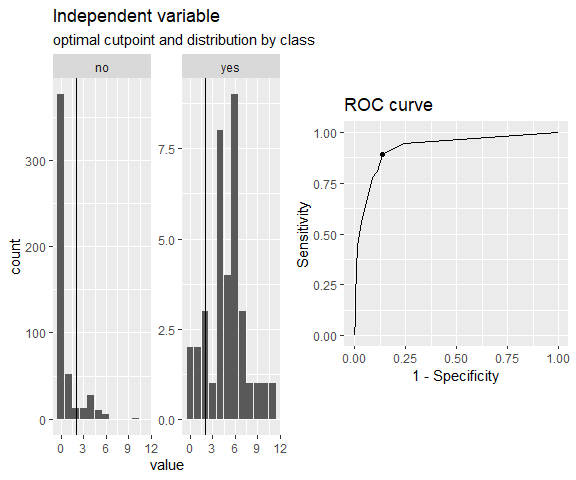When considering the optimality of a cutpoint, we can only make a judgement based on the sample at hand. Thus, the estimated cutpoint may not be optimal within the population or on unseen data, which is why we sometimes put the “optimal” in quotation marks.

cutpointr makes assumptions about the direction of the dependency between class and x, if direction and / or pos_class or neg_class are not specified. The same result as above can be achieved by manually defining direction and the positive / negative classes which is slightly faster, since the classes and direction don’t have to be determined:

opt_cut <- cutpointr(suicide, dsi, suicide, direction = ">=", pos_class = "yes",
neg_class = "no", method = maximize_metric, metric = youden)

opt_cut is a data frame that returns the input data and the ROC curve (and optionally the bootstrap results) in a nested tibble. Methods for summarizing and plotting the data and results are included (e.g. summary, plot, plot_roc, plot_metric)

To inspect the optimization, the function of metric values per cutpoint can be plotted using plot_metric, if an optimization function was used that returns a metric column in the roc_curve column. For example, the maximize_metric and minimize_metric functions do so:

plot_metric(opt_cut)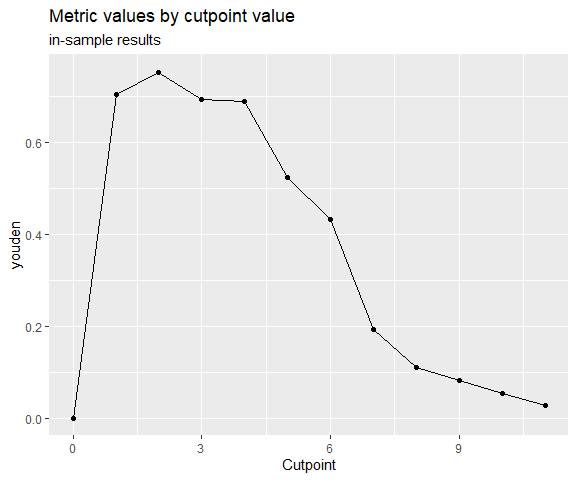Predictions for new data can be made using predict:

predict(opt_cut, newdata = data.frame(dsi = 0:5))
##  "no"  "no"  "yes" "yes" "yes" "yes"

## Features

• Calculation of optimal cutpoints in binary classification tasks
• Tidy output, integrates well with functions from the tidyverse
• Functions for plotting ROC curves, metric distributions and more
• Bootstrapping for simulating the cutpoint variability and for obtaining out-of-bag estimates of various metrics (as a form of internal validation) with optional parallelisation
• Multiple methods for calculating cutpoints
• Multiple metrics can be chosen for maximization / minimization
• Tidyeval

# Calculating cutpoints

## Method functions for cutpoint estimation

The included methods for calculating cutpoints are:

• maximize_metric: Maximize the metric function
• minimize_metric: Minimize the metric function
• maximize_loess_metric: Maximize the metric function after LOESS smoothing
• minimize_loess_metric: Minimize the metric function after LOESS smoothing
• maximize_spline_metric: Maximize the metric function after spline smoothing
• minimize_spline_metric: Minimize the metric function after spline smoothing
• maximize_gam_metric: Maximize the metric function after smoothing via Generalized Additive Models
• minimize_gam_metric: Minimize the metric function after smoothing via Generalized Additive Models
• maximize_boot_metric: Bootstrap the optimal cutpoint when maximizing a metric
• minimize_boot_metric: Bootstrap the optimal cutpoint when minimizing a metric
• oc_manual: Specify the cutoff value manually
• oc_mean: Use the sample mean as the “optimal” cutpoint
• oc_median: Use the sample median as the “optimal” cutpoint
• oc_youden_kernel: Maximize the Youden-Index after kernel smoothing the distributions of the two classes
• oc_youden_normal: Maximize the Youden-Index parametrically assuming normally distributed data in both classes

## Metric functions

The included metrics to be used with the minimization and maximization methods are:

• accuracy: Fraction correctly classified
• abs_d_sens_spec: The absolute difference of sensitivity and specificity
• abs_d_ppv_npv: The absolute difference between positive predictive value (PPV) and negative predictive value (NPV)
• roc01: Distance to the point (0,1) on ROC space
• cohens_kappa: Cohen’s Kappa
• sum_sens_spec: sensitivity + specificity
• sum_ppv_npv: The sum of positive predictive value (PPV) and negative predictive value (NPV)
• prod_sens_spec: sensitivity * specificity
• prod_ppv_npv: The product of positive predictive value (PPV) and negative predictive value (NPV)
• youden: Youden- or J-Index = sensitivity + specificity - 1
• odds_ratio: (Diagnostic) odds ratio
• risk_ratio: risk ratio (relative risk)
• p_chisquared: The p-value of a chi-squared test on the confusion matrix
• cost_misclassification: The sum of the misclassification cost of false positives and false negatives. Additional arguments: cost_fp, cost_fn
• total_utility: The total utility of true / false positives / negatives. Additional arguments: utility_tp, utility_tn, cost_fp, cost_fn
• F1_score: The F1-score (2 * TP) / (2 * TP + FP + FN)
• metric_constrain: Maximize a selected metric given a minimal value of another selected metric
• sens_constrain: Maximize sensitivity given a minimal value of specificity
• spec_constrain: Maximize specificity given a minimal value of sensitivity
• acc_constrain: Maximize accuracy given a minimal value of sensitivity

Furthermore, the following functions are included which can be used as metric functions but are more useful for plotting purposes, for example in plot_cutpointr, or for defining new metric functions: tp, fp, tn, fn, tpr, fpr, tnr, fnr, false_omission_rate, false_discovery_rate, ppv, npv, precision, recall, sensitivity, and specificity.

The inputs to the arguments method and metric are functions so that user-defined functions can easily be supplied instead of the built-in ones.

## Separate subgroups and bootstrapping

Cutpoints can be separately estimated on subgroups that are defined by a third variable, gender in this case. Additionally, if boot_runs is larger zero, cutpointr will carry out the usual cutpoint calculation on the full sample, just as before, and additionally on boot_runs bootstrap samples. This offers a way of gauging the out-of-sample performance of the cutpoint estimation method. If a subgroup is given, the bootstrapping is carried out separately for every subgroup which is also reflected in the plots and output.

set.seed(12)
opt_cut_b <- cutpointr(suicide, dsi, suicide, boot_runs = 1000)
opt_cut_b
## # A tibble: 1 x 16
##   direction optimal_cutpoint method          sum_sens_spec      acc sensitivity
##   <chr>                <dbl> <chr>                   <dbl>    <dbl>       <dbl>
## 1 >=                       2 maximize_metric       1.75179 0.864662    0.888889
##   specificity      AUC pos_class neg_class prevalence outcome predictor
##         <dbl>    <dbl> <fct>     <fct>          <dbl> <chr>   <chr>
## 1    0.862903 0.923779 yes       no         0.0676692 suicide dsi
##   data               roc_curve                 boot
##   <list>             <list>                    <list>
## 1 <tibble [532 x 2]> <roc_cutpointr [13 x 10]> <tibble [1,000 x 23]>

The returned object has the additional column boot which is a nested tibble that includes the cutpoints per bootstrap sample along with the metric calculated using the function in metric and various default metrics. The metrics are suffixed by _b to indicate in-bag results or _oob to indicate out-of-bag results:

## Smoothing via Generalized Additive Models for selecting a cutpoint

In a similar fashion, the function of metric values per cutpoint can be smoothed using Generalized Additive Models with smooth terms. Internally, mgcv::gam carries out the smoothing which can be customized via the arguments formula and optimizer, see help("gam", package = "mgcv"). Most importantly, the GAM can be specified by altering the default formula, for example the smoothing function could be configured to apply cubic regression splines ("cr") as the smooth term. As the suicide data has only very few unique cutpoints, it is not very suitable for showcasing the GAM smoothing, so we will use two classes of the iris data here. In this case, the purely empirical method and the GAM smoothing lead to identical cutpoints, but in practice the GAM smoothing tends to be more robust, especially with larger data. An attractive feature of the GAM smoothing is that the default values tend to work quite well and usually require no tuning, eliminating researcher degrees of freedom.

library(ggplot2)
exdat <- iris
exdat <- exdat[exdat$Species != "setosa", ] opt_cut <- cutpointr(exdat, Petal.Length, Species, method = minimize_gam_metric, formula = m ~ s(x.sorted, bs = "cr"), metric = abs_d_sens_spec) ## Assuming the positive class is virginica ## Assuming the positive class has higher x values plot_metric(opt_cut)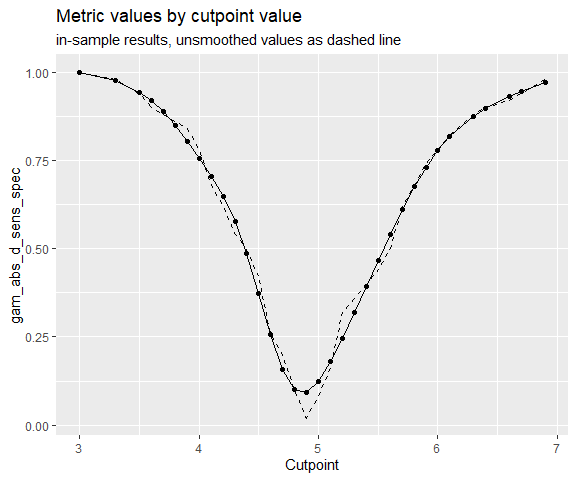## Spline smoothing for selecting a cutpoint Again in the same fashion the function of metric values per cutpoint can be smoothed using smoothing splines. By default, the number of knots is automatically chosen using the cutpoint_knots function. That function uses stats::.nknots.smspl, which is the default in stats::smooth.spline to pick the number of knots. Alternatively, the number of knots can be set manually and also the other smoothing parameters of stats::smooth.spline can be set as desired. For details see ?maximize_spline_metric. opt_cut <- cutpointr(suicide, dsi, suicide, gender, method = minimize_spline_metric, spar = 0.4, metric = misclassification_cost, cost_fp = 1, cost_fn = 10) ## Assuming the positive class is yes ## Assuming the positive class has higher x values ## nknots: 10 ## nknots: 10 plot_metric(opt_cut)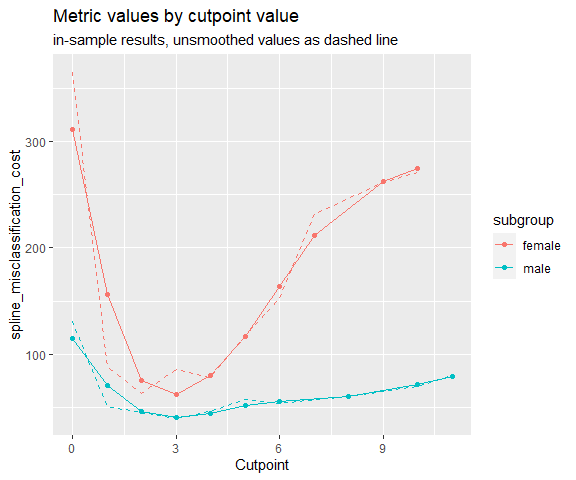### Parametric method assuming normality The Normal method in oc_youden_normal is a parametric method for maximizing the Youden-Index or equivalently the sum of $$Se$$ and $$Sp$$. It relies on the assumption that the predictor for both the negative and positive observations is normally distributed. In that case it can be shown that $c^* = \frac{(\mu_P \sigma_N^2 - \mu_N \sigma_P^2) - \sigma_N \sigma_P \sqrt{(\mu_N - \mu_P)^2 + (\sigma_N^2 - \sigma_P^2) log(\sigma_N^2 / \sigma_P^2)}}{\sigma_N^2 - \sigma_P^2}$ where the negative class is normally distributed with $$\sim N(\mu_N, \sigma_N^2)$$ and the positive class independently normally distributed with $$\sim N(\mu_P, \sigma_P^2)$$ provides the optimal cutpoint $$c^*$$ that maximizes the Youden-Index. If $$\sigma_N$$ and $$\sigma_P$$ are equal, the expression can be simplified to $$c^* = \frac{\mu_N + \mu_P}{2}$$. However, the oc_youden_normal method in cutpointr always assumes unequal standard deviations. Since this method does not select a cutpoint from the observed predictor values, it is questionable which values for $$Se$$ and $$Sp$$ should be reported. Here, the Youden-Index can be calculated as $J = \Phi(\frac{c^* - \mu_N}{\sigma_N}) - \Phi(\frac{c^* - \mu_P}{\sigma_P})$ if the assumption of normality holds. However, since there exist several methods that do not select cutpoints from the available observations and to unify the reporting of metrics for these methods, cutpointr reports all metrics, e.g. $$Se$$ and $$Sp$$, based on the empirical observations. cutpointr(suicide, dsi, suicide, gender, method = oc_youden_normal) ## Assuming the positive class is yes ## Assuming the positive class has higher x values ## # A tibble: 2 x 18 ## subgroup direction optimal_cutpoint method sum_sens_spec acc ## <chr> <chr> <dbl> <chr> <dbl> <dbl> ## 1 female >= 2.47775 oc_youden_normal 1.71618 0.895408 ## 2 male >= 3.17226 oc_youden_normal 1.54453 0.864286 ## sensitivity specificity AUC pos_class neg_class prevalence outcome ## <dbl> <dbl> <dbl> <fct> <fct> <dbl> <chr> ## 1 0.814815 0.901370 0.944647 yes no 0.0688776 suicide ## 2 0.666667 0.877863 0.861747 yes no 0.0642857 suicide ## predictor grouping data roc_curve boot ## <chr> <chr> <list> <list> <lgl> ## 1 dsi gender <tibble [392 x 2]> <roc_cutpointr [11 x 9]> NA ## 2 dsi gender <tibble [140 x 2]> <roc_cutpointr [11 x 9]> NA ### Nonparametric kernel method A nonparametric alternative is the Kernel method [@fluss_estimation_2005]. Here, the empirical distribution functions are smoothed using the Gaussian kernel functions $$\hat{F}_N(t) = \frac{1}{n} \sum^n_{i=1} \Phi(\frac{t - y_i}{h_y})$$ and $$\hat{G}_P(t) = \frac{1}{m} \sum^m_{i=1} \Phi(\frac{t - x_i}{h_x})$$ for the negative and positive classes respectively. Following Silverman’s plug-in “rule of thumb” the bandwidths are selected as $$h_y = 0.9 * min\{s_y, iqr_y/1.34\} * n^{-0.2}$$ and $$h_x = 0.9 * min\{s_x, iqr_x/1.34\} * m^{-0.2}$$ where $$s$$ is the sample standard deviation and $$iqr$$ is the inter quartile range. It has been demonstrated that AUC estimation is rather insensitive to the choice of the bandwidth procedure [@faraggi_estimation_2002] and thus the plug-in bandwidth estimator has also been recommended for cutpoint estimation. The oc_youden_kernel function in cutpointr uses a Gaussian kernel and the direct plug-in method for selecting the bandwidths. The kernel smoothing is done via the bkde function from the KernSmooth package [@wand_kernsmooth:_2013]. Again, there is a way to calculate the Youden-Index from the results of this method [@fluss_estimation_2005] which is $\hat{J} = max_c \{\hat{F}_N(c) - \hat{G}_N(c) \}$ but as before we prefer to report all metrics based on applying the cutpoint that was estimated using the Kernel method to the empirical observations. cutpointr(suicide, dsi, suicide, gender, method = oc_youden_kernel) ## Assuming the positive class is yes ## Assuming the positive class has higher x values ## # A tibble: 2 x 18 ## subgroup direction optimal_cutpoint method sum_sens_spec acc ## <chr> <chr> <dbl> <chr> <dbl> <dbl> ## 1 female >= 1.18128 oc_youden_kernel 1.80812 0.885204 ## 2 male >= 1.31636 oc_youden_kernel 1.58694 0.807143 ## sensitivity specificity AUC pos_class neg_class prevalence outcome ## <dbl> <dbl> <dbl> <fct> <fct> <dbl> <chr> ## 1 0.925926 0.882192 0.944647 yes no 0.0688776 suicide ## 2 0.777778 0.809160 0.861747 yes no 0.0642857 suicide ## predictor grouping data roc_curve boot ## <chr> <chr> <list> <list> <lgl> ## 1 dsi gender <tibble [392 x 2]> <roc_cutpointr [11 x 9]> NA ## 2 dsi gender <tibble [140 x 2]> <roc_cutpointr [11 x 9]> NA # Additional features ## Calculating only the ROC curve When running cutpointr, a ROC curve is by default returned in the column roc_curve. This ROC curve can be plotted using plot_roc. Alternatively, if only the ROC curve is desired and no cutpoint needs to be calculated, the ROC curve can be created using roc() and plotted using plot_cutpointr. The roc function, unlike cutpointr, does not determine direction, pos_class or neg_class automatically. roc_curve <- roc(data = suicide, x = dsi, class = suicide, pos_class = "yes", neg_class = "no", direction = ">=") auc(roc_curve) ##  0.9237791 head(roc_curve) ## # A tibble: 6 x 9 ## x.sorted tp fp tn fn tpr tnr fpr fnr ## <dbl> <dbl> <dbl> <int> <int> <dbl> <dbl> <dbl> <dbl> ## 1 Inf 0 0 496 36 0 1 0 1 ## 2 11 1 0 496 35 0.0278 1 0 0.972 ## 3 10 2 1 495 34 0.0556 0.998 0.00202 0.944 ## 4 9 3 1 495 33 0.0833 0.998 0.00202 0.917 ## 5 8 4 1 495 32 0.111 0.998 0.00202 0.889 ## 6 7 7 1 495 29 0.194 0.998 0.00202 0.806 plot_roc(roc_curve)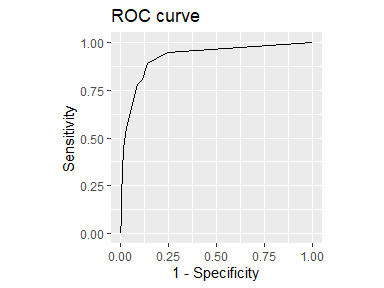## Midpoints So far - which is the default in cutpointr - we have considered all unique values of the predictor as possible cutpoints. An alternative could be to use a sequence of equidistant values instead, for example in the case of the suicide data all integers in $$[0, 10]$$. However, with very sparse data and small intervals between the candidate cutpoints (i.e. a ‘dense’ sequence like seq(0, 10, by = 0.01)) this leads to the uninformative evaluation of large ranges of cutpoints that all result in the same metric value. A more elegant alternative, not only for the case of sparse data, that is supported by cutpointr is the use of a mean value of the optimal cutpoint and the next highest (if direction = ">=") or the next lowest (if direction = "<=") predictor value in the data. The result is an optimal cutpoint that is equal to the cutpoint that would be obtained using an infinitely dense sequence of candidate cutpoints and is thus usually more efficient computationally. This behavior can be activated by setting use_midpoints = TRUE, which is the default. If we use this setting, we obtain an optimal cutpoint of 1.5 for the complete sample on the suicide data instead of 2 when maximizing the sum of sensitivity and specificity. Assume the following small data set: dat <- data.frame(outcome = c("neg", "neg", "neg", "pos", "pos", "pos", "pos"), pred = c(1, 2, 3, 8, 11, 11, 12)) Since the distance of the optimal cutpoint (8) to the next lowest observation (3) is rather large we arrive at a range of possible cutpoints that all maximize the metric. In the case of this kind of sparseness it might for example be desirable to classify a new observation with a predictor value of 4 as belonging to the negative class. If use_midpoints is set to TRUE, the mean of the optimal cutpoint and the next lowest observation is returned as the optimal cutpoint, if direction is >=. The mean of the optimal cutpoint and the next highest observation is returned as the optimal cutpoint, if direction = "<=". opt_cut <- cutpointr(dat, x = pred, class = outcome, use_midpoints = TRUE) ## Assuming the positive class is pos ## Assuming the positive class has higher x values plot_x(opt_cut)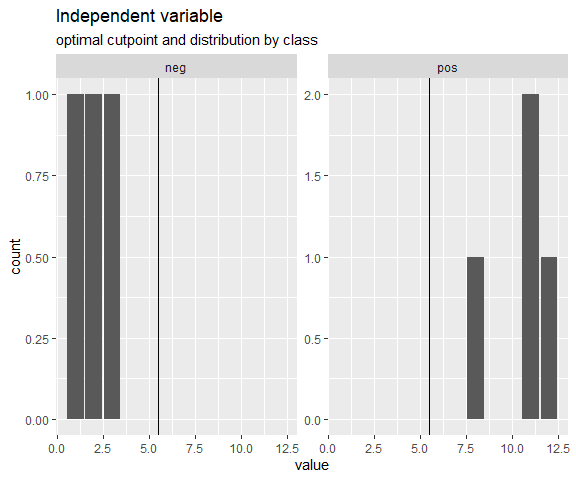A simulation demonstrates more clearly that setting use_midpoints = TRUE avoids biasing the cutpoints. To simulate the bias of the metric functions, the predictor values of both classes were drawn from normal distributions with constant standard deviations of 10, a constant mean of the negative class of 100 and higher mean values of the positive class that are selected in such a way that optimal Youden-Index values of 0.2, 0.4, 0.6, and 0.8 result in the population. Samples of 9 different sizes were drawn and the cutpoints that maximize the Youden-Index were estimated. The simulation was repeated 10000 times. As can be seen by the mean error, use_midpoints = TRUE eliminates the bias that is introduced by otherwise selecting the value of an observation as the optimal cutpoint. If direction = ">=", as in this case, the observation that represents the optimal cutpoint is the highest possible cutpoint that leads to the optimal metric value and thus the biases are positive. The methods oc_youden_normal and oc_youden_kernel are always unbiased, as they don’t select a cutpoint based on the ROC-curve or the function of metric values per cutpoint. ## ## Attaching package: 'dplyr' ## The following objects are masked from 'package:stats': ## ## filter, lag ## The following objects are masked from 'package:base': ## ## intersect, setdiff, setequal, union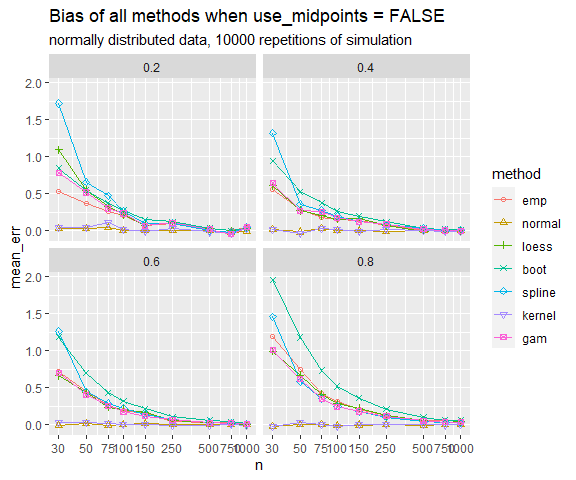## Finding all cutpoints with acceptable performance By default, most packages only return the “best” cutpoint and disregard other cutpoints with quite similar performance, even if the performance differences are minuscule. cutpointr makes this process more explicit via the tol_metric argument. For example, if all cutpoints are of interest that achieve at least an accuracy within 0.05 of the optimally achievable accuracy, tol_metric can be set to 0.05 and also those cutpoints will be returned. In the case of the suicide data and when maximizing the sum of sensitivity and specificity, empirically the cutpoints 2 and 3 lead to quite similar performances. If tol_metric is set to 0.05, both will be returned. opt_cut <- cutpointr(suicide, dsi, suicide, metric = sum_sens_spec, tol_metric = 0.05, break_ties = c) ## Assuming the positive class is yes ## Assuming the positive class has higher x values ## Multiple optimal cutpoints found, applying break_ties. library(tidyr) opt_cut %>% select(optimal_cutpoint, sum_sens_spec) %>% unnest(cols = c(optimal_cutpoint, sum_sens_spec)) ## # A tibble: 2 x 2 ## optimal_cutpoint sum_sens_spec ## <dbl> <dbl> ## 1 2 1.75 ## 2 1 1.70 ## Manual and mean / median cutpoints Using the oc_manual function the optimal cutpoint will not be determined based on, for example, a metric but is instead set manually using the cutpoint argument. This is useful for supplying and evaluating cutpoints that were found in the literature or in other external sources. The oc_manual function could also be used to set the cutpoint to the sample mean using cutpoint = mean(data$x). However, this may introduce bias into the bootstrap validation procedure, since the actual mean of the population is not known and thus the mean to be used as the cutpoint should be automatically determined in every resample. To do so, the oc_mean and oc_median functions can be used.

set.seed(100)
opt_cut_manual <- cutpointr(suicide, dsi, suicide, method = oc_manual,
cutpoint = mean(suicide\$dsi), boot_runs = 1000)
set.seed(100)
opt_cut_mean <- cutpointr(suicide, dsi, suicide, method = oc_mean, boot_runs = 1000)

## Nonstandard evaluation via tidyeval

The arguments to cutpointr do not need to be enclosed in quotes. This is possible thanks to nonstandard evaluation of the arguments, which are evaluated on data.

Functions that use nonstandard evaluation are often not suitable for programming with. The use of nonstandard evaluation may lead to scoping problems and subsequent obvious as well as possibly subtle errors. cutpointr uses tidyeval internally and accordingly the same rules as for programming with dplyr apply. Arguments can be unquoted with !!:

myvar <- "dsi"
cutpointr(suicide, !!myvar, suicide)

## ROC curve and optimal cutpoint for multiple variables

Alternatively, we can map the standard evaluation version cutpointr to the column names. If direction and / or pos_class and neg_class are unspecified, these parameters will automatically be determined by cutpointr so that the AUC values for all variables will be $$> 0.5$$.

We could do this manually, e.g. using purrr::map, but to make this task more convenient multi_cutpointr can be used to achieve the same result. It maps multiple predictor columns to cutpointr, by default all numeric columns except for the class column.

mcp <- multi_cutpointr(suicide, class = suicide, pos_class = "yes",
use_midpoints = TRUE, silent = TRUE)
summary(mcp)
## Method: maximize_metric
## Predictor: age, dsi
## Outcome: suicide
##
## Predictor: age
## --------------------------------------------------------------------------------
##  direction    AUC   n n_pos n_neg
##         <= 0.5257 532    36   496
##
##  optimal_cutpoint sum_sens_spec    acc sensitivity specificity tp fn  fp tn
##              55.5        1.1154 0.1992      0.9722      0.1431 35  1 425 71
##
## Predictor summary:
##     Data Min. 5% 1st Qu. Median    Mean 3rd Qu.   95% Max.      SD NAs
##  Overall   18 19      24   28.0 34.1259   41.25 65.00   83 15.0542   0
##       no   18 19      24   28.0 34.2218   41.25 65.50   83 15.1857   0
##      yes   18 18      22   27.5 32.8056   41.25 54.25   69 13.2273   0
##
## Predictor: dsi
## --------------------------------------------------------------------------------
##  direction    AUC   n n_pos n_neg
##         >= 0.9238 532    36   496
##
##  optimal_cutpoint sum_sens_spec    acc sensitivity specificity tp fn fp  tn
##               1.5        1.7518 0.8647      0.8889      0.8629 32  4 68 428
##
## Predictor summary:
##     Data Min.   5% 1st Qu. Median   Mean 3rd Qu.  95% Max.     SD NAs
##  Overall    0 0.00       0      0 0.9211       1 5.00   11 1.8527   0
##       no    0 0.00       0      0 0.6331       0 4.00   10 1.4122   0
##      yes    0 0.75       4      5 4.8889       6 9.25   11 2.5498   0

## Accessing data, roc_curve, and boot

The object returned by cutpointr is of the classes cutpointr, tbl_df, tbl, and data.frame. Thus, it can be handled like a usual data frame. The columns data, roc_curve, and boot consist of nested data frames, which means that these are list columns whose elements are data frames. They can either be accessed using [ or by using functions from the tidyverse. If subgroups were given, the output contains one row per subgroup and the function that accesses the data should be mapped to every row or the data should be grouped by subgroup.

set.seed(123)
opt_cut_b_g <- cutpointr(suicide, dsi, suicide, gender, boot_runs = 1000)
# Using dplyr and tidyr
library(tidyr)
opt_cut_b_g %>%
group_by(subgroup) %>%
select(subgroup, boot) %>%
unnest(cols = boot) %>%
summarise(sd_oc_boot = sd(optimal_cutpoint),
m_oc_boot  = mean(optimal_cutpoint),
m_acc_oob  = mean(acc_oob))
## # A tibble: 2 x 4
##   subgroup sd_oc_boot m_oc_boot m_acc_oob
##   <chr>         <dbl>     <dbl>     <dbl>
## 1 female        0.766      2.17     0.880
## 2 male          1.51       2.92     0.806

## Adding metrics to the result of cutpointr() or roc()

By default, the output of cutpointr includes the optimized metric and several other metrics. The add_metric function adds further metrics. Here, we’re adding the negative predictive value (NPV) and the positive predictive value (PPV) at the optimal cutpoint per subgroup:

cutpointr(suicide, dsi, suicide, gender, metric = youden, silent = TRUE) %>%
select(subgroup, optimal_cutpoint, youden, ppv, npv)
## # A tibble: 2 x 5
##   subgroup optimal_cutpoint   youden      ppv      npv
##   <chr>               <dbl>    <dbl>    <dbl>    <dbl>
## 1 female                  2 0.808118 0.367647 0.993827
## 2 male                    3 0.625106 0.259259 0.982301

In the same fashion, additional metric columns can be added to a roc_cutpointr object:

roc(data = suicide, x = dsi, class = suicide, pos_class = "yes",
neg_class = "no", direction = ">=") %>%
select(x.sorted, tp, fp, tn, fn, cohens_kappa, F1_score) %>%
head()
## # A tibble: 6 x 7
##   x.sorted    tp    fp    tn    fn cohens_kappa F1_score
##      <dbl> <dbl> <dbl> <int> <int>        <dbl>    <dbl>
## 1      Inf     0     0   496    36       0        0
## 2       11     1     0   496    35       0.0506   0.0541
## 3       10     2     1   495    34       0.0931   0.103
## 4        9     3     1   495    33       0.138    0.15
## 5        8     4     1   495    32       0.182    0.195
## 6        7     7     1   495    29       0.301    0.318

## User-defined functions

### method

User-defined functions can be supplied to method, which is the function that is responsible for returning the optimal cutpoint. To define a new method function, create a function that may take as input(s):

• data: A data.frame or tbl_df
• x: (character) The name of the predictor variable
• class: (character) The name of the class variable
• metric_func: A function for calculating a metric, e.g. accuracy. Note that the method function does not necessarily have to accept this argument
• pos_class: The positive class
• neg_class: The negative class
• direction: ">=" if the positive class has higher x values, "<=" otherwise
• tol_metric: (numeric) In the built-in methods, all cutpoints will be returned that lead to a metric value in the interval [m_max - tol_metric, m_max + tol_metric] where m_max is the maximum achievable metric value. This can be used to return multiple decent cutpoints and to avoid floating-point problems.
• use_midpoints: (logical) In the built-in methods, if TRUE (default FALSE) the returned optimal cutpoint will be the mean of the optimal cutpoint and the next highest observation (for direction = “>”) or the next lowest observation (for direction = “<”) which avoids biasing the optimal cutpoint.
• ...: Further arguments that are passed to metric or that can be captured inside of method

The function should return a data frame or tibble with one row, the column optimal_cutpoint, and an optional column with an arbitrary name with the metric value at the optimal cutpoint.

For example, a function for choosing the cutpoint as the mean of the independent variable could look like this:

mean_cut <- function(data, x, ...) {
oc <- mean(data[[x]])
return(data.frame(optimal_cutpoint = oc))
}

If a method function does not return a metric column, the default sum_sens_spec, the sum of sensitivity and specificity, is returned as the extra metric column in addition to accuracy, sensitivity and specificity.

Some method functions that make use of the additional arguments (that are captured by ...) are already included in cutpointr, see the list at the top. Since these functions are arguments to cutpointr their code can be accessed by simply typing their name, see for example oc_youden_normal.

### metric

User defined metric functions can be used as well. They are mainly useful in conjunction with method = maximize_metric, method = minimize_metric, or one of the other minimization and maximization functions. In case of a different method function metric will only be used as the main out-of-bag metric when plotting the result. The metric function should accept the following inputs as vectors:

• tp: Vector of true positives
• fp: Vector of false positives
• tn: Vector of true negatives
• fn: Vector of false negatives
• ...: Further arguments

The function should return a numeric vector, a matrix, or a data.frame with one column. If the column is named, the name will be included in the output and plots. Avoid using names that are identical to the column names that are by default returned by cutpointr, as such names will be prefixed by metric_ in the output. The inputs (tp, fp, tn, and fn) are vectors. The code of the included metric functions can be accessed by simply typing their name.

For example, this is the misclassification_cost metric function:

misclassification_cost
## function (tp, fp, tn, fn, cost_fp = 1, cost_fn = 1, ...)
## {
##     misclassification_cost <- cost_fp * fp + cost_fn * fn
##     misclassification_cost <- matrix(misclassification_cost,
##         ncol = 1)
##     colnames(misclassification_cost) <- "misclassification_cost"
##     return(misclassification_cost)
## }
## <bytecode: 0x00000000247b08c0>
## <environment: namespace:cutpointr>

# Plotting

cutpointr includes several convenience functions for plotting data from a cutpointr object. These include:

• plot_cutpointr: General purpose plotting function for cutpointr or roc_cutpointr objects
• plot_cut_boot: Plot the bootstrapped distribution of optimal cutpoints
• plot_metric: If maximize_metric or minimize_metric was used this function plots all possible cutoffs on the x-axis vs. the respective metric values on the y-axis. If bootstrapping was run, a confidence interval based on the bootstrapped distribution of metric values at each cutpoint can be displayed. To display no confidence interval set conf_lvl = 0.
• plot_metric_boot: Plot the distribution of out-of-bag metric values
• plot_precision_recall: Plot the precision recall curve
• plot_sensitivity_specificity: Plot all cutpoints vs. sensitivity and specificity
• plot_roc: Plot the ROC curve
• plot_x: Plot the distribution of the predictor variable
plot_cut_boot(opt_cut_b_g)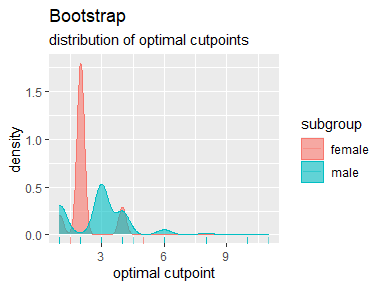plot_metric(opt_cut_b_g, conf_lvl = 0.9)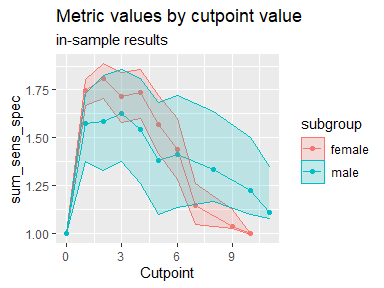plot_metric_boot(opt_cut_b_g)
## Warning: Removed 12 rows containing non-finite values (stat_density).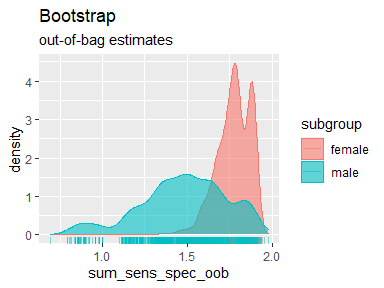plot_precision_recall(opt_cut_b_g)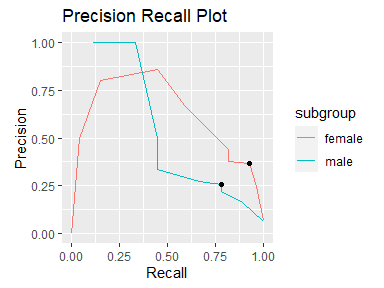plot_sensitivity_specificity(opt_cut_b_g)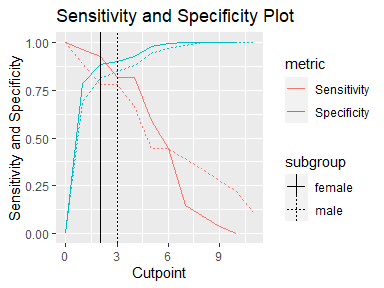plot_roc(opt_cut_b_g)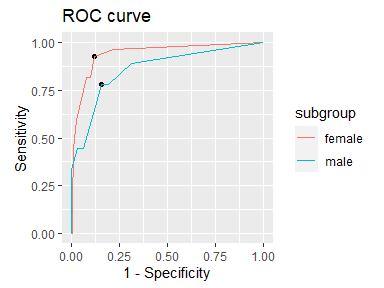All plot functions, except for the standard plot method that returns a composed plot, return ggplot objects than can be further modified. For example, changing labels, title, and the theme can be achieved this way:

p <- plot_x(opt_cut_b_g)
p + ggtitle("Distribution of dsi") + theme_minimal() + xlab("Depression score")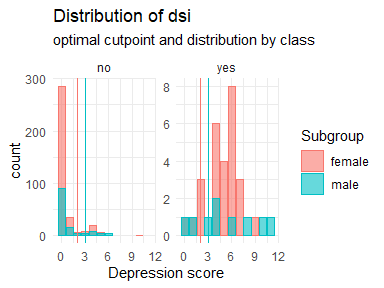## Flexible plotting function

Using plot_cutpointr any metric can be chosen to be plotted on the x- or y-axis and results of cutpointr() as well as roc() can be plotted. If a cutpointr object is to be plotted, it is thus irrelevant which metric function was chosen for cutpoint estimation. Any metric that can be calculated based on the ROC curve can be subsequently plotted as only the true / false positives / negatives over all cutpoints are needed. That way, not only the above plots can be produced, but also any combination of two metrics (or metric functions) and / or cutpoints. The built-in metric functions as well as user-defined functions or anonymous functions can be supplied to xvar and yvar. If bootstrapping was run, confidence intervals can be plotted around the y-variable. This is especially useful if the cutpoints, available in the cutpoints function, are placed on the x-axis. Note that confidence intervals can only be correctly plotted if the values of xvar are constant across bootstrap samples. For example, confidence intervals for TPR by FPR (a ROC curve) cannot be plotted easily, as the values of the false positive rate vary per bootstrap sample.

plot_cutpointr(opt_cut_b, xvar = cutpoints, yvar = sum_sens_spec, conf_lvl = 0.9)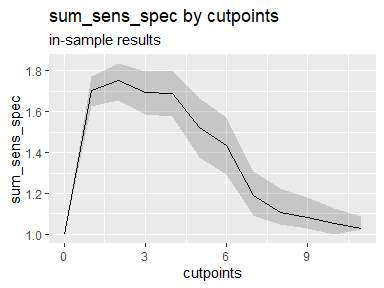plot_cutpointr(opt_cut_b, xvar = fpr, yvar = tpr, aspect_ratio = 1, conf_lvl = 0)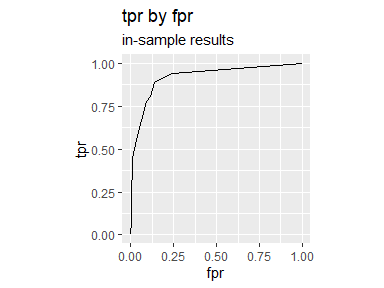plot_cutpointr(opt_cut_b, xvar = cutpoint, yvar = tp, conf_lvl = 0.9) + geom_point()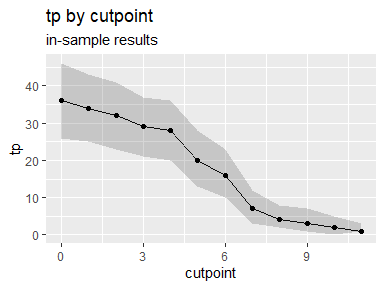## Manual plotting

Since cutpointr returns a data.frame with the original data, bootstrap results, and the ROC curve in nested tibbles, these data can be conveniently extracted and plotted manually. The relevant nested tibbles are in the columns data, roc_curve and boot. The following is an example of accessing and plotting the grouped data.

opt_cut_b_g %>%
select(data, subgroup) %>%
unnest(cols = data) %>%
ggplot(aes(x = suicide, y = dsi)) +
geom_boxplot(alpha = 0.3) + facet_grid(~subgroup)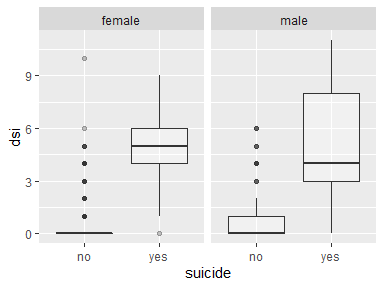# Benchmarks

To offer a comparison to established solutions, cutpointr will be benchmarked against optimal.cutpoints from the OptimalCutpoints package, ThresholdROC and custom functions based on the ROCR and pROC packages. By generating data of different sizes the benchmarks will offer a comparison of the scalability of the different solutions.

Using prediction and performance from the ROCR package and roc from the pROC package, we can write functions for computing the cutpoint that maximizes the sum of sensitivity and specificity. pROC has a built-in function to optimize a few metrics:

# Return cutpoint that maximizes the sum of sensitivity and specificiy
# ROCR package
rocr_sensspec <- function(x, class) {
pred <- ROCR::prediction(x, class)
perf <- ROCR::performance(pred, "sens", "spec")
sens <- slot(perf, "y.values")[]
spec <- slot(perf, "x.values")[]
cut <- slot(perf, "alpha.values")[]
cut[which.max(sens + spec)]
}

# pROC package
proc_sensspec <- function(x, class) {
r <- pROC::roc(class, x, algorithm = 2, levels = c(0, 1), direction = "<")
pROC::coords(r, "best", ret="threshold", transpose = FALSE)
}

The benchmarking will be carried out using the microbenchmark package and randomly generated data. The values of the x predictor variable are drawn from a normal distribution which leads to a lot more unique values than were encountered before in the suicide data. Accordingly, the search for an optimal cutpoint is much more demanding, if all possible cutpoints are evaluated.

Benchmarks are run for sample sizes of 100, 1000, 1e4, 1e5, 1e6, and 1e7. For low sample sizes cutpointr is slower than the other solutions. While this should be of low practical importance, cutpointr scales more favorably with increasing sample size. The speed disadvantage in small samples that leads to the lower limit of around 25ms is mainly due to the nesting of the original data and the results that makes the compact output of cutpointr possible. This observation is emphasized by the fact that cutpointr::roc is quite fast also in small samples. For sample sizes > 1e5 cutpointr is a little faster than the function based on ROCR and pROC. Both of these solutions are generally faster than OptimalCutpoints and ThresholdROC with the exception of small samples. OptimalCutpoints and ThresholdROC had to be excluded from benchmarks with more than 1e4 observations due to high memory requirements and/or excessive run times, rendering the use of these packages in larger samples impractical.

# ROCR package
rocr_roc <- function(x, class) {
pred <- ROCR::prediction(x, class)
perf <- ROCR::performance(pred, "sens", "spec")
return(NULL)
}

# pROC package
proc_roc <- function(x, class) {
r <- pROC::roc(class, x, algorithm = 2, levels = c(0, 1), direction = "<")
return(NULL)
}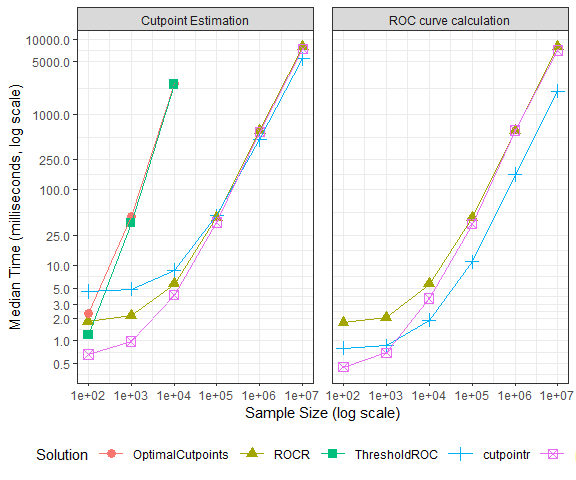n task OptimalCutpoints ROCR ThresholdROC cutpointr pROC
1e+02 Cutpoint Estimation 2.288702 1.812802 1.194301 4.5018015 0.662101
1e+03 Cutpoint Estimation 45.056801 2.176401 36.239852 4.8394010 0.981001
1e+04 Cutpoint Estimation 2538.612001 5.667101 2503.801251 8.5662515 4.031701
1e+05 Cutpoint Estimation NA 43.118751 NA 45.3845010 37.150151
1e+06 Cutpoint Estimation NA 607.023851 NA 465.0032010 583.095000
1e+07 Cutpoint Estimation NA 7850.258700 NA 5467.3328010 7339.356101
1e+02 ROC curve calculation NA 1.732651 NA 0.7973505 0.447701
1e+03 ROC curve calculation NA 2.035852 NA 0.8593010 0.694802
1e+04 ROC curve calculation NA 5.662151 NA 1.8781510 3.658050
1e+05 ROC curve calculation NA 42.820852 NA 11.0992510 35.329301
1e+06 ROC curve calculation NA 612.471901 NA 159.8100505 610.433700
1e+07 ROC curve calculation NA 7806.385452 NA 2032.6935510 7081.897251